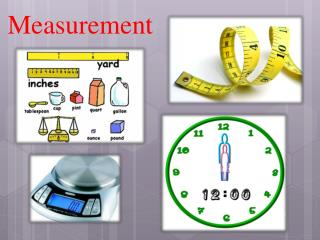DownloadDownload PresentationMeasurement

# Measurement

Download Presentation## Measurement

- - - - - - - - - - - - - - - - - - - - - - - - - - - E N D - - - - - - - - - - - - - - - - - - - - - - - - - - -
##### Presentation Transcript

1. Measurement

2. Measurement In this chapter we will explore the following concepts: 1. Measurement of a physical parameter 2. Units, systems of units 3. Basic units in mechanics 4. Changing units

3. Stating a Measurement In every measurement there is a • Number followed by a • Unit from measuring device

4. Standards of Measurement When we measure, we use a measuring tool to compare some dimension of an object to a standard.

5. Some Tools for Measurement

6. Basic & Derived Quantities • Basic Quantity  Must be defined in terms of a standard (meter, kilogram, second). • Derived Quantity  Defined in terms of combinations of basic quantities • Unit of speed = meter/second = m/s

7. International System of Units: In this system the units for the base quantities are: Unit of Length: the Meter A meter is a unit of length, currently defined as the distance light travels within 1/299782458th of a second.

8. Time Unit –second (s) The time needed for a cesium-133 atom to perform 9,192,631,770 complete oscillations

9. Volume Unit – the Liter (L) Volume is the amount of space occupied by a substance. S.I unit of volume is liter (L). The milliliter (ml) is commonly used for measuring smaller volumes of fluids. 1 gallon = 4 quarts 1 liter (L) = 1.06 quart 1 quart = 946 mL 1 L = 10 deciliters (dL) = 100 centilliters (cL) = 1000 milliliters (mL)

10. Mass The mass of an object is a measure of the quantity of material it contains. In the SI system, the mass unit is the kilogram (kg). In the metric system, the mass unit is the gram (g). Compare to US weight units: 1 kg = 2.20 lbs 1 lb = 454 grams

11. Temperature Scales • The degree of hotness or coldness is called temperature. • The S.I unit of temperature is kelvin • Other Scales: • Celsius (C) scale • Fahrenheit (F) scale • 1 C = 1.8 F

12. Other Systems of Units • CGS centimeter-gram-second • FPS foot-pound-second

13. Learning Check From the previous slide, state the tool (s) you would use to measure A. temperature ____________________ B. volume ____________________ ____________________ C. time ____________________ D. weight ____________________

14. From the previous slide, state the tool (s) you would use to measure A. Temperaturethermometer B.Volumemeasuring cup, graduated cylinder C.Timewatch, clocks D. Weightspring balance

15. Unit conversions We will work exclusively in SI (MKS) units!

16. Unit Conversion

17. Learning Check What are some S.I units that are used to measure each of the following? A. length B. volume C. weight D. temperature

18. Some possible answers are A. length inch, foot, yard, mile B. volume cup, teaspoon, gallon, pint, quart C. weight ounce, pound (lb), ton D. temperature °F

19. Units in the Metric System • length meter m • volume liter L • mass gram g • temperature Celsius °C

20. Learning Check Identify the measurement in metric units. A. John’s height is 1) 1.5 yards2) 6 feet 3) 2 meters B. The volume of Pepsi in a big bottle is 1) 1 liters 2) 1 quart 3) 2 pints C. The mass of a lemon is 1) 12 ounces2) 145 grams 3) 0.6 pounds

21. Solution A. John’s height is 3) 2 meters B. The volume of Pepsi in a big bottle is 1) 1 liter C. The mass of a lemon is 2) 145 grams

22. Changing Units

23. Units of Volume: • The SI unit of volume is the cubic meter (m)3. • A more convenient unit of volume for everyday use is the liter, a non-SI unit. • A liter (L) is the volume of a cube that is 10 centimeters (10 cm) along each edge (10 cm  10 cm  10 cm = 1000 cm3 = 1 L).

24. Units and Quantities Scientists commonly use two equivalent units of temperature, the degree Celsius and the kelvin. Units of Temperature

25. Units and Quantities • The joule (J) is the SI unit of energy. • One calorie (cal) is the quantity of heat that raises the temperature of 1 g of pure water by 1°C.

26. Section Quiz. 1. Which of the following is not a base SI unit? • gram • meter • second • mole

27. Section Quiz. • A temperature of 30 degrees Celsius is equivalent to • 303 K. • 300 K. • 243 K. • 247 K.

28. THE END